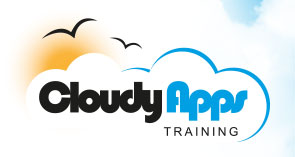# DAV101 - Introduction to Data Analysis and Visualisation with R

## Course Background

This course provides an intensive introduction to the use of R for data analyis and visualisation. Starting with an overview of R and its uses it then goes on to explore data management and retrieval from within R, import and export of data between R and spreadsheet packages such as Excel, the R programming language and the accessing relational databases from R. The course also explores a variety of data visualisation techniques available in R as well as the implementation of various programs in R to automate much of the work of data analysis and visualisation. It is a hands-on course filled with real data and examples, case studies, and in-class mini projects. It should be considered as a foundational course that lays the foundation for more advanced data analysis and visualisation projects involving the analysis and mining of large and complex data sets.

## Course Prerequisites and Target Audience

Strudents are expected to have a basic knowledge of statistics and programming, such as might have been gained in as part of an undergraduate satistics course in e.g. Economics, Business Studies or Psychology. The level of programming assumed is such as might have been gained by writing e.g. shell programs or simple python scripts, or basic VBA programming.

## Course Outline

• Overview and Introduction to R
• R as a computing environment
• R interface, session, and language overview
• The RStudio IDE
• Data Manipulation
• Basic data types and operations
• Data Frames
• Importing, saving, exporting, and re-using data
• Standard R functions for numbers, factors, text, and dates
• Vector-oriented computation
• Sorting, ranking, and printing
• R as a Programming Language
• Data types and variables
• Loops and conditions
• Functions - implementation and use
• Object-oriented aspects of R programming – lists and classes
• Code optimisation and efficient data processing
• Exploratory Data Analysis
• Basic data summary functions
• Tables and cross-tabulations
• Graphics programming in R
• Basic plots and charts
• Modifying plots, labels, titles, and advanced customization
• Trellis graphics
• Saving graphics and graphic formats
• Statistics and Modeling in R
• Overview of modeling and statistical data analysis
• Basic statistical tests, power, and sample size functions
• Regression and analysis of variance
• Basic data mining functions
• Modular programming in R
• Object oriented programming and the object oriented features of R
• Controlling object evaluation and R's scoping rules
• Understanding base functions such as with, subset and transform
• Capturing user input without evaluating it
• Controllng when and where R evaluates expressions and calls
• R’s rules for dynamic and lexical scoping
• Writing code that modifies code
• R functions as first class objects
• Creating anonymous functions
• Writing closures – functions that return functions
• Building higher-order functions – functions that take other functions as input
• Working with lists of functions
• S3 and S4 generic functions and classes
• R5 - Reference classes
• Operating system functions and system calls
• Working with R from the command line
• R and SQL
• Overview of relational databases and SQL
• Using sqldf to write SQL commands to extract data from a data frame
• Using the DBI package to interact with relational databases from R
• Data visualisation in R
• Overview of ggplot and plyr
• Creating informative scatterplots
• Adding extra variables with aesthetics (e.g. color, shape and size) or facetting.
• Create graphics for large data
• Histograms and bar charts for displaying distributional summaries
• Boxplots
• Scatterplots variations that overcome the over-plotting problems associated with large data.
• Designing and critiquing a graphic